# Recursion CMSC 201 Recursion Vs Iteration Recursion and

• Slides: 20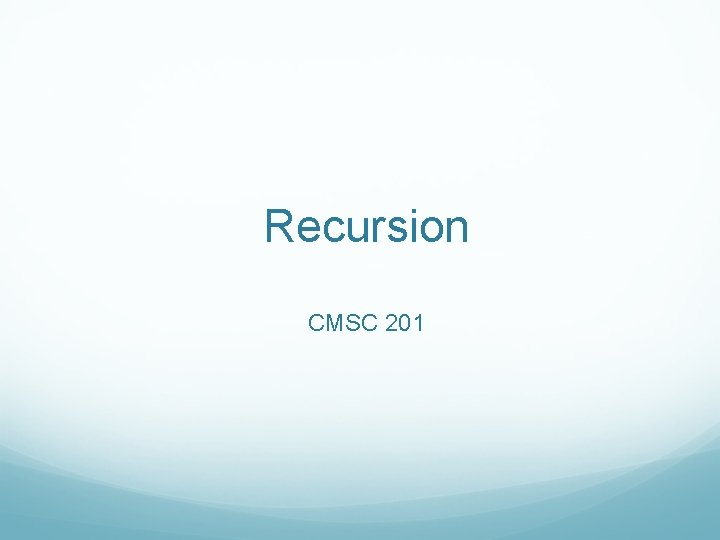Recursion CMSC 201Recursion Vs. Iteration Recursion and iteration are both methods of solving problems. An iterative solution to a problem is anything involving loops. Everything we’ve done so far has been iterative. Anything that can be done iteratively can be done recursively.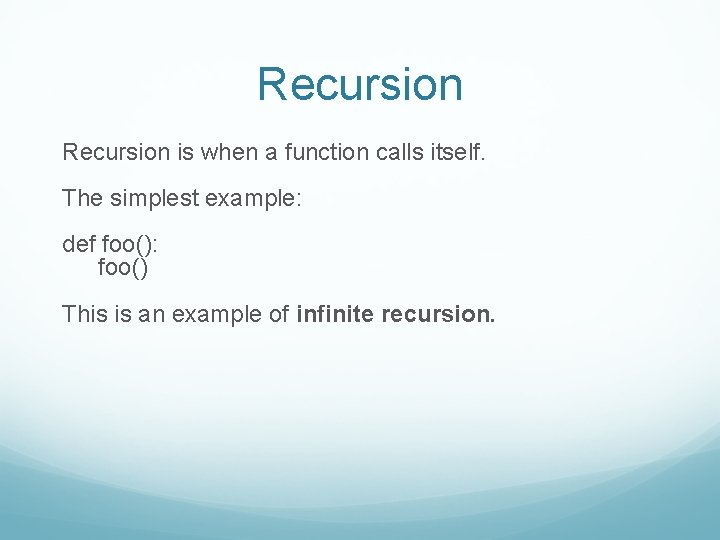Recursion is when a function calls itself. The simplest example: def foo(): foo() This is an example of infinite recursion.Recursion Infinite recursion: def foo(): foo() Infinite loop: while True: passRecursion We can get loop like behavior by passing arguments! def foo(i): print(i) foo(i-1) What does this do? How might we get it to terminate?Recursion def foo(i): if(i > 0): return print(i) foo(i-1) Is equivalent to: while a > 0: print(a) a=a+1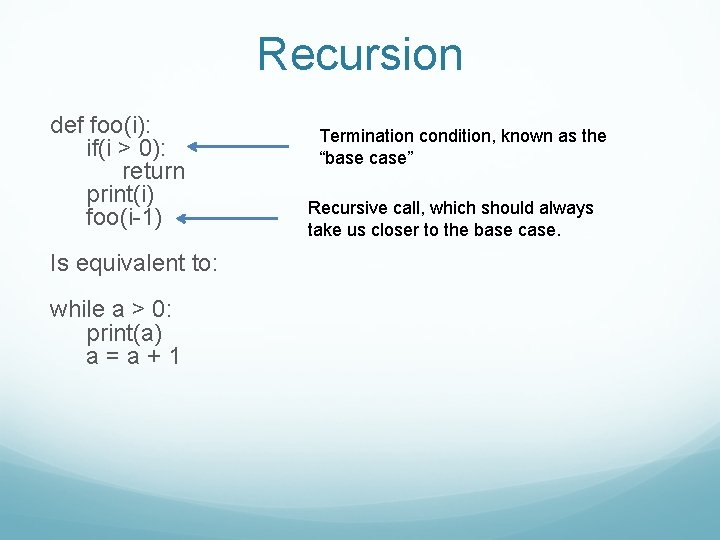Recursion def foo(i): if(i > 0): return print(i) foo(i-1) Is equivalent to: while a > 0: print(a) a=a+1 Termination condition, known as the “base case” Recursive call, which should always take us closer to the base case.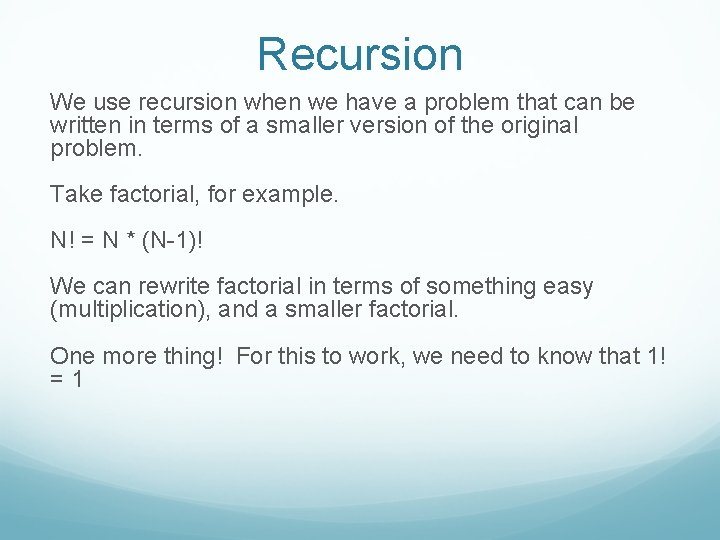Recursion We use recursion when we have a problem that can be written in terms of a smaller version of the original problem. Take factorial, for example. N! = N * (N-1)! We can rewrite factorial in terms of something easy (multiplication), and a smaller factorial. One more thing! For this to work, we need to know that 1! =1Factorial def factorial(num): if(num == 1): return 1 return num * factorial(num-1)Helper Functions Sometimes in recursion we have to pass information as arguments. When we do this, we use a helper function to keep the user from sending incorrect arguments (this will make more sense in the next slide).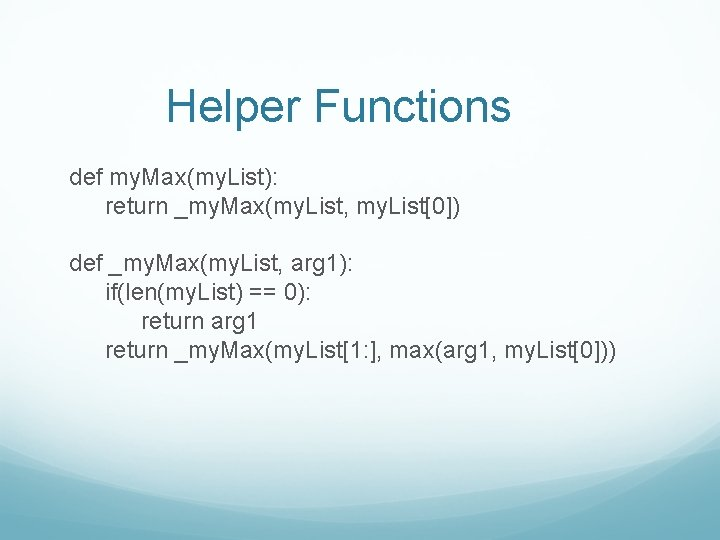Helper Functions def my. Max(my. List): return _my. Max(my. List, my. List) def _my. Max(my. List, arg 1): if(len(my. List) == 0): return arg 1 return _my. Max(my. List[1: ], max(arg 1, my. List))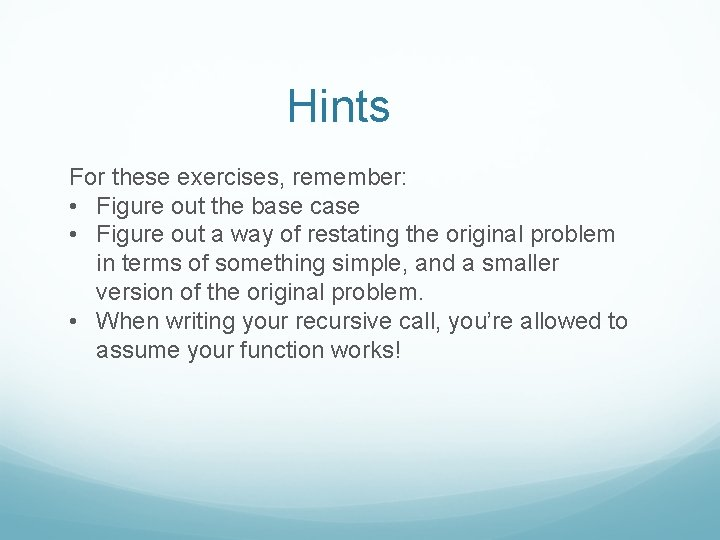Hints For these exercises, remember: • Figure out the base case • Figure out a way of restating the original problem in terms of something simple, and a smaller version of the original problem. • When writing your recursive call, you’re allowed to assume your function works!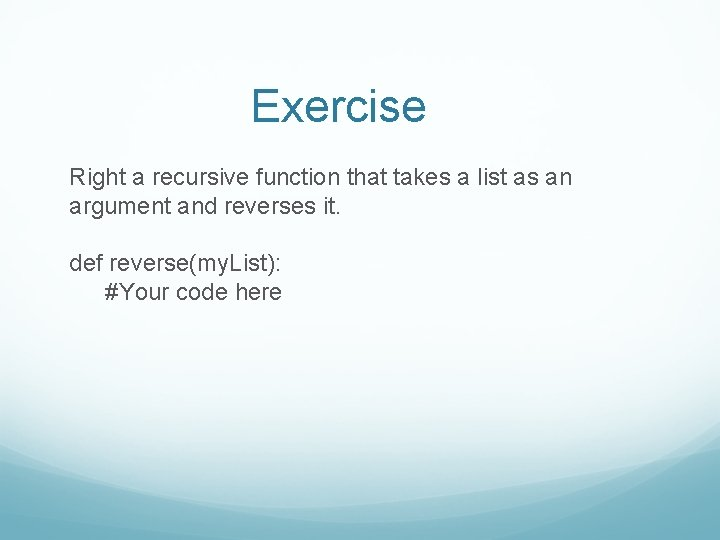Exercise Right a recursive function that takes a list as an argument and reverses it. def reverse(my. List): #Your code hereExercise Write a recursive function that takes a list as an argument and reverses it. def reverse(my. List): if(len(my. List) == 1): return my. List return [my. List[-1]] + reverse(my. List[: -1])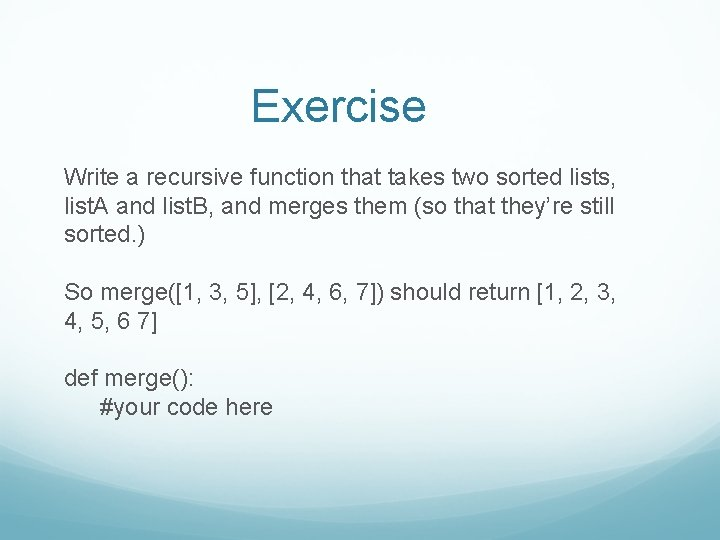Exercise Write a recursive function that takes two sorted lists, list. A and list. B, and merges them (so that they’re still sorted. ) So merge([1, 3, 5], [2, 4, 6, 7]) should return [1, 2, 3, 4, 5, 6 7] def merge(): #your code hereExercise def merge(list. A, list. B): if(len(list. A) == 0): return list. B if(len(list. B) == 0): return list. A if(list. A > list. B): return [list. B] + merge(list. A, list. B[1: ]) else: return [list. A] + merge(list. A[1: ], list. B)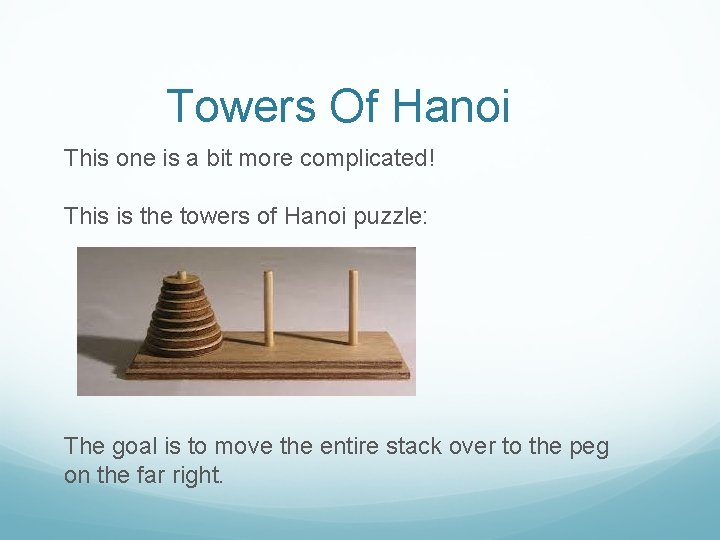Towers Of Hanoi This one is a bit more complicated! This is the towers of Hanoi puzzle: The goal is to move the entire stack over to the peg on the far right.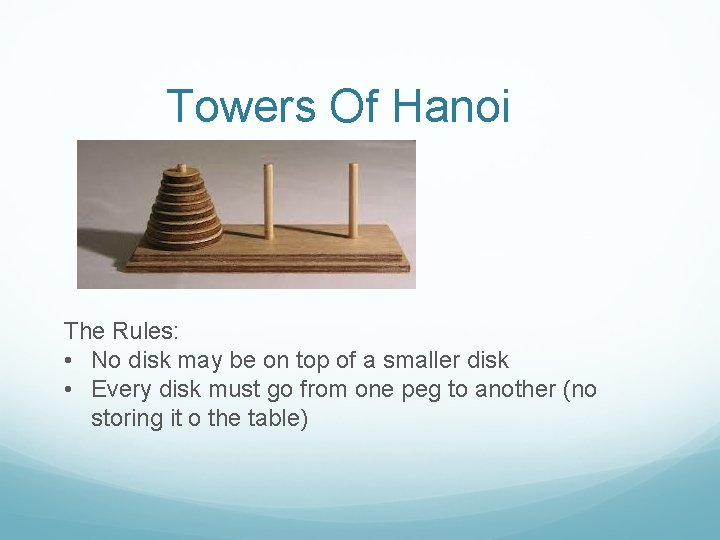Towers Of Hanoi The Rules: • No disk may be on top of a smaller disk • Every disk must go from one peg to another (no storing it o the table)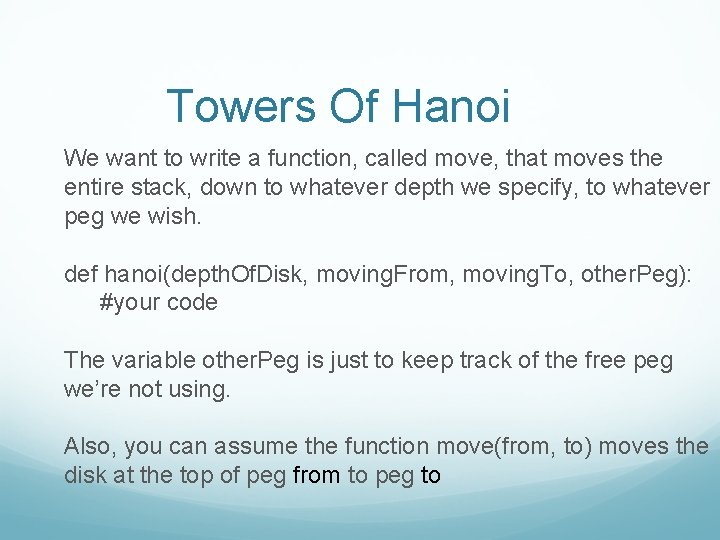Towers Of Hanoi We want to write a function, called move, that moves the entire stack, down to whatever depth we specify, to whatever peg we wish. def hanoi(depth. Of. Disk, moving. From, moving. To, other. Peg): #your code The variable other. Peg is just to keep track of the free peg we’re not using. Also, you can assume the function move(from, to) moves the disk at the top of peg from to peg to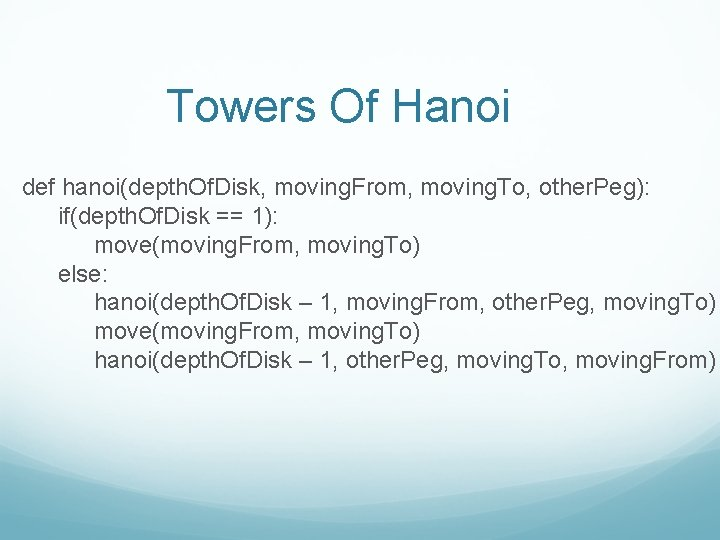Towers Of Hanoi def hanoi(depth. Of. Disk, moving. From, moving. To, other. Peg): if(depth. Of. Disk == 1): move(moving. From, moving. To) else: hanoi(depth. Of. Disk – 1, moving. From, other. Peg, moving. To) move(moving. From, moving. To) hanoi(depth. Of. Disk – 1, other. Peg, moving. To, moving. From)